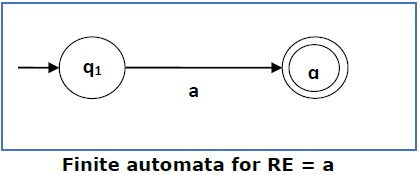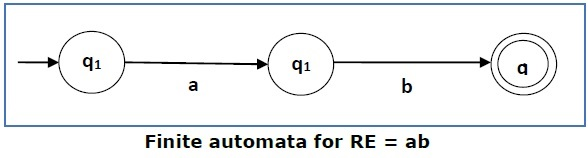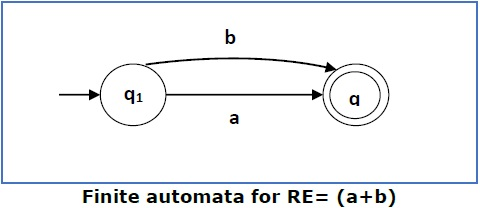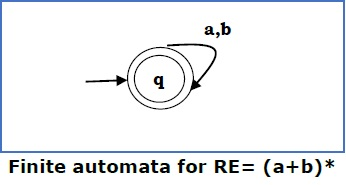# From a Regular expression to an NFA

We can use Thompson’s Construction to find out a Finite Automaton from a Regular Expression. We will reduce the regular expression into smallest regular expressions and converting these to NFA#### Some basic RA expressions are the following −

Case 1 − For a regular expression ‘a’, we can construct the following FA −Case 2 − For a regular expression ‘ab’, we can construct the following FA −Case 3 − For a regular expression (a+b), we can construct the following FA −Case 4 − For a regular expression (a+b)*, we can construct the following FA −### NEXT – Conversion from NFA to DFA